# [3D基础]理解计算机3D图形学中的坐标系变换

要谈坐标系变换，那么坐标系有哪些呢？依次有:物体坐标系，世界坐标系，相机坐标系，投影坐标系以及屏幕坐标系.我要讨论的就是这些坐标系间的转换。
这些坐标系不是凭空而来，他们都是为了完成计算机3D图形学最最最基本的目标而出现.
计算机3D图形学最最最基本的目标就是:将构建好的3D物体显示在2D屏幕坐标上.
初看好像就是将最初的物体坐标系转换到屏幕坐标系就可以了呀，为什么多出了世界坐标系,相机坐标系，投影坐标 系。这是因为:在一个大世界里有多个物体，而每个物体都有自己的坐标系，如何表述这些物体间相对的关系，这个多出了世界坐标系；如果只需要看到这个世界其 中一部分，这里就多出了相机坐标系；至于投影坐标系那是因为直接将3D坐标转换为屏幕坐标是非常复杂的(因为它们不仅维度不同,度量不同（屏幕坐标一般都 是像素为单位,3D空间中我们可以现实世界的米，厘米为单位），XY的方向也不同，在2D空间时还要进行坐标系变换),所以先将3D坐标降维到2D坐标, 然后2D坐标转换到屏幕坐标。

为什么会有左手坐标系与右手坐标系之分？
在3D空间(没错！就是3D)中，所有2D坐标系是等价的（就是通过一系列的仿射变换，可以互相转换）
而3D坐标系不是等价的，通过仿射变换，是无法将左手坐标系转换到右手坐标系；也就是说，物体坐标系用的就是左手坐标系，世界坐标系用的是右手 坐标系，那么物体可能就是不会是我们所希望的样子了，可能是倒立的，也可能是背对着我们的，所以我们要区分左手坐标系与右手坐标系。也许在4D空间，左右 手坐标系就可以互相变换了吧。
进入正题吧:
首先讨论的是物体坐标系->世界坐标系
前面说了为了描述多个物体间相对的关系，这里引进了世界坐标系,所以世界坐标系是个参考坐标系。
这一步的目的将所有的物体的点都转移到世界坐标系，这里主要涉及的是旋转，缩放，平移等。
不过我将详细说明为何及如何用矩阵来描述这些变换。
例:如果有两个坐标系C与C`, C`是C绕Z轴旋转θ得到的。下面是各坐标轴的变换：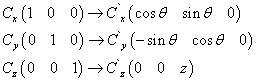如果是C坐标系的点P（x, y, z），而在C`的表示就是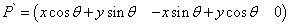这时该如何建立矩阵呢? 答案就是区分你用的是行向量还是列向量.也许有人会问为什么不区分是左手坐标系还是右手坐标系呢？因为C可以变换到C`,那么他们一定是同在左手坐标系或右手坐标系,变换只能在可以互相转换的坐标系之间进行。
如果你用的是行向量：由于行向量只能左乘矩阵(注意乘与乘以的区别)
所以矩阵形式应该是这样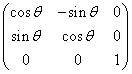只有这样，在左乘矩阵时才能得到上面P`的形式。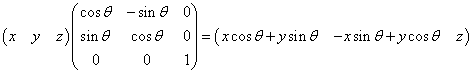如果你用的是列向量: 由于列向量只能右乘矩阵(注意乘与乘以的区别)
所以矩阵形式应该是这样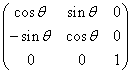只有这样，在右乘矩阵时才能得到上面P`的形式。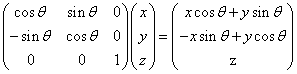至于如何旋转，缩放，平移我不在多说。
…………………………………觉得自己好像跑题了.还好这两个坐标系变换很简单。
我们再讨论世界坐标系->相机坐标系
引进相机的目的就是只需看到世界的一部分，而哪些是可以在相机里看到的，就需要进行筛选。将物体转换到相机坐标系，这样相机坐标系进行筛选时就会简单很多。这里的重点是构建相机坐标系。
物体坐标系，世界坐标系是美工在绘制时就定义好了的。而相机坐标系是需要程序实时构建的。（当然这是通常情况下，如果你要建立一个世界，这个世界都是围绕 你转，要实时改变所有物体坐标系，固定相机坐标系（其实这时候相机坐标系就是世界坐标系），建立一个地心说的世界，我也没办法，你的思维也太不一样了。）
如何构建相机坐标系呢？首先我们要明确目标：我们是要构建3D坐标系(好像是废话)，三个坐标轴要互相垂直(也好像是废话).
我们一般用UVN相机。例如:D3D的D3DXMatrixLookAtLH，D3DXMatrixLookAtRH，OGL的gluLookAt(右手坐标系).
如何建立呢UVN相机呢? 我们就要利用叉积这个工具了：两个不平行，不重叠的向量的叉积可以得到与这两个向量互相垂直的向量。
如果有了相机的位置与目标的位置那么我们可以确定一个Z轴（有人问为什么是Z轴，因为物体的远与近我们就习惯用Z值来表示的）。求Z轴时要注意 是左手坐标系还是右手坐标系，左右手坐标系XY轴方向相同时，Z轴的方向相反。所以左手坐标系是目标位置减去相机位置，而右手坐标系则是相机位置减去目标 位置。记得normalize
这是我们要得到X与Y轴了。如何求X，Y轴呢？
一般方法是：
1、选择一个临时Y轴，
2、对临时Y 与Z 轴进行叉积求得一个X轴
3、X轴再与Z轴进行叉积，得到一个Y轴。
有了XYZ就可以求出旋转的相机矩阵了。
如何选择一个Y轴呢？大多数情况下是（0，1，0），但是如果是相机位置E与目标位置T垂直,即（E-T=（0，+/-1，0）时），这时就不能用(0,1,0)了， 因为两个平行向量的叉积是零向量，所以我们就要另选一个Y轴。
但是我觉得我们可以改变方法。
如果不能选Y轴，我们就选择一个临时X轴，这个临时Ｘ轴就是（1，0，0）。
然后再对临时X轴与Z轴进行叉积求得一个Y轴。
最后Y轴再与Z轴进行叉积，得到X轴。
这样可以得到XYZ轴。
最后再根据行向量与列向量建立相机矩阵，再进行平移。

相机坐标系->投影坐标系.
投影的目的就是：降维.
两种投影方式：正交投影与透视投影.
在我们TEAM中易颖已经写了，我就不多说了，大家去看他的文章。

投影坐标系->屏幕坐标系
这是最简单的。2D坐标变换。也不多说。

1、用多维数列表示低维空间坐标,加深理解齐次坐标表示法。

齐次坐标表示法可以方便地运算，同时形状不变。[x,y,z,0]表示一个无穷的点。

2、透视投影变换公式可以看成两个矩阵的乘积，其中一个做透视变换，另外一个作正投影

保留的z'值的确切含义：指的是在完全作完透视投影变换之前，仅作透视投影之后的一条线.

它的几何意义见李建平《计算机图形学原理教程》第44页。

3、左手和右手坐标系的坐标转换

“视点坐标系与一般的物体所在的世界坐标系不同，它遵循左手法则，即左手大拇指指向Z正轴，与之垂直的四个手指指向X正轴，四指弯曲90度的方向是Y正轴。而世界坐标系遵循右手法则的。”

4、视点坐标系的透视变换公式很重要！！王飞著计算机图形学书65

5z'值的确切含义：指的是在完全作完透视投影变换之前，仅作透视投影之后的一条线

[xw,yw,zw,1]****[xe ye ze 1]=[cos*xw-sin*yw+l   zw+m   2sin*xw+2cos*yw+2n-d   (sin*xw+cos*yw+n/d]

=[(cos*xw-sin*yw+l)*d/(sin*xw+cos*yw+n   (zw+m)*d/(sin*xw+cos*yw+n)   2sin*xw+2cos*yw+2n-d*d/(sin*xw+cos*yw+n)    1 ]

Xe= (cos*xw-sin*yw+l)*d/(sin*xw+cos*yw+n)

Ye=(zw+m)*d/(sin*xw+cos*yw+n)

Ze=(2sin*xw+2cos*yw+2*n-d)*d/(sin*xw+cos*yw+n)

xe:=trunc((cos(angle)*eee[ii][k].X-sin(angle)*eee[ii][k].Y+l)*d/(sin(angle)*eee[ii][k].X+cos(angle)*eee[ii][k].Y+n));

ye:=trunc((hhh[ii][k]+m)*d/(sin(angle)*eee[ii][k].X+cos(angle)*eee[ii][k].Y+n)); //透视变换

//ze可以考虑使用作为消隐

Ze:=trunc((2*sin(angle)*xw+2*cos(angle)*yw+2*n-d)*d/(sin(angle)*xw+cos(angle)*yw+n));

Xe= (cos*xw-sin*yw+l)*d/(sin*xw+cos*yw+n)          1

Ye=(zw+m)*d/(sin*xw+cos*yw+n)                         2

Ze=2sin*xw+2cos*yw+2n-d*d/(sin*xw+cos*yw+n) 3

1）除以（2）得

Xe/Ye= (cos*xw-sin*yw+l)/ (zw+m)

(Xe*(zw+m))/Ye=cos*xw-sin*yw+l

(Xe*(zw+m))/(Ye* cos)=xw-tan*yw+l/cosXw=(Xe*(zw+m))/ (Ye* cos) - (ye*l)/(Ye* cos)+( sin*yw*ye)/ (Ye* cos)

Xw=(Xe*(zw+m)+ sin*yw*ye- ye*l)/ (Ye* cos)            (4)

Ye*(sin*xw+cos*yw+n)= (zw+m)*d

Ye*(sin*xw)+ye* cos*yw+n*ye=(zw+m)*d

Ye* sin*(Xe*(zw+m)+ sin*yw*ye- ye*l)/ (Ye* cos)+ye* cos*yw+n*ye=(zw+m)*d

sin*(Xe*(zw+m)+ sin*yw*ye- ye*l)/ cos+ye* cos*yw+n*ye=(zw+m)*d

tan*(Xe*zw+xe*m+ sin*yw*ye- ye*l) +ye* cos*yw+n*ye=(zw+m)*d

tan*Xe*zw+xe*m *tan- ye*l*tan+ sin*tan*yw*ye+ye* cos*yw+n*ye=(zw+m)*d

(sin*tan*ye+ye* cos)*yw+ tan*Xe*zw+xe*m *tan- ye*l*tan+n*ye=(zw+m)*d

Yw=[(zw+m)*d- tan*Xe*zw- xe*m *tan+ ye*l*tan- n*ye]/ (sin*tan*ye+ye* cos) 5

Xw=(Xe*(zw+m)+ sin*yw*ye- ye*l)/ (Ye* cos)            (4)

posted on 2010-12-06 14:19 wrh 阅读(916) 评论(0)  编辑 收藏 引用### 导航

 < 2011年2月 >
303112345
6789101112
13141516171819
20212223242526
272812345
6789101112

• 随笔 - 242
• 文章 - 11
• 评论 - 52
• 引用 - 0

•